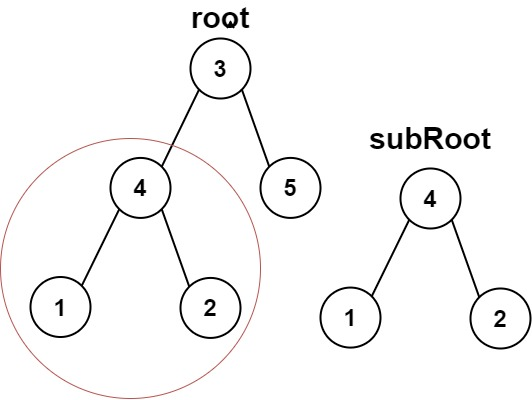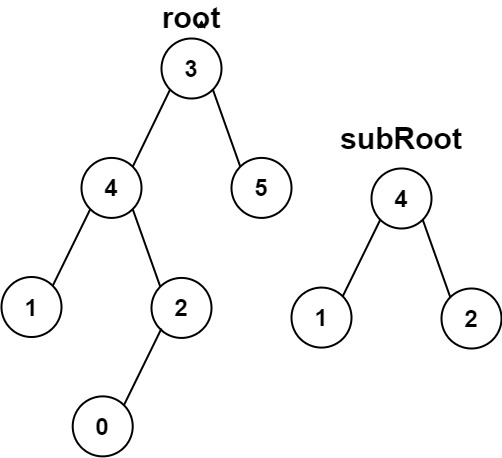# GeetCode Hub

Given the roots of two binary trees `root` and `subRoot`, return `true` if there is a subtree of `root` with the same structure and node values of` subRoot` and `false` otherwise.

A subtree of a binary tree `tree` is a tree that consists of a node in `tree` and all of this node's descendants. The tree `tree` could also be considered as a subtree of itself.

Example 1:```Input: root = [3,4,5,1,2], subRoot = [4,1,2]
Output: true
```

Example 2:```Input: root = [3,4,5,1,2,null,null,null,null,0], subRoot = [4,1,2]
Output: false
```

Constraints:

• The number of nodes in the `root` tree is in the range `[1, 2000]`.
• The number of nodes in the `subRoot` tree is in the range `[1, 1000]`.
• `-104 <= root.val <= 104`
• `-104 <= subRoot.val <= 104`

/** * Definition for a binary tree node. * public class TreeNode { * int val; * TreeNode left; * TreeNode right; * TreeNode() {} * TreeNode(int val) { this.val = val; } * TreeNode(int val, TreeNode left, TreeNode right) { * this.val = val; * this.left = left; * this.right = right; * } * } */ class Solution { public boolean isSubtree(TreeNode root, TreeNode subRoot) { } }